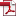• KSII Transactions on Internet and Information Systems
Monthly Online Journal (eISSN: 1976-7277)

The alternative Method to Finish Modular Exponentiation and Point Multiplication Processes

Vol. 15, No. 7, July 31, 202110.3837/tiis.2021.07.017, Download Paper (Free):Abstract

The aim of this paper is to propose the alternative algorithm to finish the process in public key cryptography. In general, the proposed method can be selected to finish both of modular exponentiation and point multiplication. Although this method is not the best method in all cases, it may be the most efficient method when the condition responds well to this approach. Assuming that the binary system of the exponent or the multiplier is considered and it is divided into groups, the binary system is in excellent condition when the number of groups is small. Each group is generated from a number of 0 that is adjacent to each other. The main idea behind the proposed method is to convert the exponent or the multiplier as the subtraction between two integers. For these integers, it is impossible that the bit which is equal to 1 will be assigned in the same position. The experiment is split into two sections. The first section is an experiment to examine the modular exponentiation. The results demonstrate that the cost of completing the modular multiplication is decreased if the number of groups is very small. In tables 7 – 9, four modular multiplications are required when there is one group, although number of bits which are equal to 0 in each table is different. The second component is the experiment to examine the point multiplication process in Elliptic Curves Cryptography. The findings demonstrate that if the number of groups is small, the costs to compute point additions are low. In tables 10 – 12, assigning one group is appeared, number of point addition is one when the multiplier of a point is an even number. However, three-point additions are required when the multiplier is an odd number. As a result, the proposed method is an alternative way that should be used when the number of groups is minimal in order to save the costs.